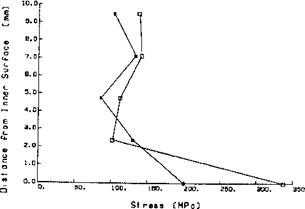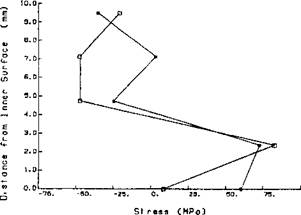Stresses, Strains and Deformations

Thermal-elastic-plastic constitutive models decompose the total strain rate ej01 into the elastic ЄуЄ, plastic due to rate

independent plasticity, thermal є* consisting of thermal expansion

and creep strain rate ej. During phase transformations additional

terms, , i. e., the strain rate volume change associated with the

transformation and єіЬр, i. e., the strain rate transformation plasticity are included:

The volume change associated with the transformation is often included as part of the thermal strain by modifying the coefficient of thermal expansion. Separation of the transformation volume change from the thermal strain allows more flexibility in the description of

the material . Oddy et al.  defined ejrp to be a function of

the stress deviator T’, relative transformation volume change A V/V, transforming phase fraction z and an internal variable 5:

i T'-» = l^LlT'(2-z)z (5-2)

4 V s

where the internal variable 5 is a yield stress as in  or deformation resistance as in  and T’ is the deviatoric stress tensor.

The incremental constitutive models used with temperature dependent material properties require the increment in the strain that occurs during the time step. Thus, for a step from time n to time n+1, the incremental form of (5-2) is:

A£,"' =~^Ґ(2-2гш-&2)Ь (5-3)

where z(n) is the fraction already transformed at the start of the

increment and Az is the amount which transforms during the increment. A more accurate method of evaluating the incremental strain is by integrating the appropriate strain rate over the increment. Equation (5-3) can also be obtained from the time derivative of (5­2), integrated over the time step using the midpoint value, assuming a piecewise constant stress.

The effect of different material models on the residual stresses is shown in Figure 2-27 for conditions resembling the case studied by Hibbitt and Marcal . Oddy et al  gives a concise description of transformation-induced plasticity (TRIP). Figure 5-6 shows the through-thickness distribution of the longitudinal (axial residual stresses on the plane of symmetry), at the mid-length of the weld, with and without transformation plasticity. A significant variation in the longitudinal stress through the thickness is evident. In both cases, the highest stress exists on the inner surface. Although the

 curves are similar in shape, with and without transformation plasticity, the magnitudes are significantly different. LONGITUDINAL (AXIAL) STRESS AT MIDLENGTHFigure 5-6: Residual longitudinal stress versus distance from inner surface, at midlength —■ including transformation plasticity, —° excluding transformation plasticity, from Oddy et al .

The magnitude of residual stresses present after welding is important in the prediction of the resistance to fracture. In this case the transverse or hoop stresses are of particular interest because they will influence the growth of axial flaws.

Figure 5-7 compares the through-thickness variation of the mid­length of the weld. The transverse stress is much smaller than the longitudinal stress. In both cases the largest values are found near, but not at the inner surface. The inclusion of transformation plasticity has made a substantial difference in the transverse stress predicted on the inner surface. The magnitude of the residual transverse stress increased when transformation plasticity was included.

 TRANSVERSE (HOOP) STRESS AT MIDLENGTHFigure 5-7: Residual transverse stress versus distance from inner surface, at midlength —■ including transformation plasticity, —° excluding transformation plasticity, from Oddy et al .

The stress-dependenee of the microstructure evolution is central to transformation induced plasticity (TRIP) in martensitic transformations. Transformation plasticity arises from 2 sources. The transformation creates local, microscopic stresses and plastic strains. The interaction of these microscopic fields with the global, macroscopic stress fields results in observable, irreversible, macroscopic plastic deformation known as the Greenwood-Johnson effect. The stress fields also influence the orientation of the shear and dilatational deformations of martensite formation. This leads to the Magee effect .

To specify a particular constitutive model we must specify the plastic potential function (or dissipation function) Ф, (5-4), and the evolution equation for state variables, (5-5):

Ф= f°'V {-}[<^+1)] (5-4)

(-+D s

m

wrt effective stress a in the limit of very low rate sensitivity m.

s = hDp-r (5-5)

where the function h governs the rate of strain hardening and dynamic softening, and the function r governs the rate of static recovery. Dp is the plastic deformation rate tensor.

The deformation gradient caused by elastic and plastic strain is defined as follows:

F = FTlrl + Vu) (5-6)

where и is the displacement vector, Vи is the displacement gradient and FTh is the thermal deformation gradient. Superscript 1 denotes the inverse of the matrix. I is the 2nd order identity tensor. In the simplest case of thermal-mechanical coupling it becomes:

FTh =a-AT I (5-7)

AT is the temperature increment for the time step and a is the thermal expansion coefficient.

The total rate of stretching is:

D = De+Dp (5-8)

Superscripts e and p denote the elastic and plastic parts of the rate of stretching, respectively.

Suppose we have a set of stress and state variables at time t{a, Sj and we also have an estimate or trial value for the same set at time x = t+At. Then a stable, accurate and efficient algorithm to compute the set of stress and state variables at time x is needed. Also a linearization of the constitutive equation is needed to compute the element stiffness that is assembled into a global stiffness matrix. The consistency of this linearization is very important to achieve robust and rapid convergence of the global linearization of momentum equation.

Assume that both the plastic and total stretching rate are constant within the time step. The time derivative of Hooke’s law [33 and 35] and Goldak et al  yields:

<f (T) = <7(0 + g[Dd(Z) - JDpdm (5-9)

and for the state variables we arrive at:

T

S(T) = S(t) + jS! d(4) (5-10)

t

We assume again that S is constant over the time step. Where:

д = elasticity tensor, 4th order a = Cauchy stress

о = convected Cauchy stress

^ = dimensionless activation volume for dislocation diffusion

In every constitutive model the set of Equations (5-9) and (5-10) is a system of nonlinear equations that must be solved for each time step, at each integration point of the space domain for each global iteration, usually using a Newton-Raphson iteration.

The isotropic constitutive model suggests that for a particular integration point the elasticity tensor д is a linear combination of the two independent elastic constants:

g = 2jLm + (K-(2/3)jU)® (5-11)

where and к are the elastic shear and bulk modulus, 91 and 1 are

the fourth and second-order identity tensors, respectively.

The two following cases of rate independent plasticity and linear viscous plasticity are obtained from the plastic potential function (5­4) by substituting rate sensitivity m-+0 and m=l, respectively. The m—>0 means that (1/m) is very large compared to 1 in the exponent in (5-4).

In the welding process, changes in stress caused by deformation are assumed to travel slowly compared to the speed of sound. So, at any instant, an observed group of material particles is approximately in static equilibrium, i. e.: inertial forces are neglected. In rate independent plasticity, viscosity is zero and viscous forces are zero. In either the Lagrangian or the Eulerian reference frame, the partial differential equation of equilibrium is, at any moment:

Э<7„

—±+f,= 0 or V-<7 + f=0 (5-12)

oxj

where f is the sum of the body forces and a is the Cauchy stress.

In the FEM formulation, Equation (5-12) is transformed and integrated over the physical domain, or a reference domain with a unique mapping to the physical domain .